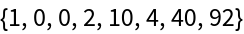Function Repository Resource:

# FindExactCover

Find row subsets of an input binary matrix that sum to 1 in each column

 ResourceFunction["FindExactCover"][mat] finds a row subset of the binary matrix mat which sums to 1 in each column. ResourceFunction["FindExactCover"][mat,All] finds all row subsets with sum equal to 1 in each column. ResourceFunction["FindExactCover"][mat,n] finds up to n row subsets with sum equal to 1 in each column.

## Details

An exact cover is a partitioning of a set of elements into disjoint subsets whose union is exactly the original set.
The exact cover (XC) problem gives an original set along with a list of allowable subsets and asks if any selection of the allowable subsets is an exact cover of the original set. It is an NP complete problem, and very similar to graph clique finding (cf. FindClique).
Here we do not deal with sets and subsets, but rather with binary matrices. The input binary matrix mat lists allowable subsets as rows and set elements as columns, by coding that the kthelement of the original set is also a member of the jth listed subset if and only if matjk=1. Thus a one's row {1,1,,1} represents a trivial exact cover.
Sometimes the terms items and options are used in place of column and row headers respectively. The exact cover problem can then be stated as follows: Choose a set of options such that every item is included in exactly one option.
If two options are not disjoint, their respective row sum will have a value exceeding 1 in at least one column. Similarly, if neither of two options includes the kth item, their respective row sum will have a 0 in the kth column. Let { j1,j2, }∈𝒪 be a set of options. It is an exact cover if and only if the respective row sum satisfies that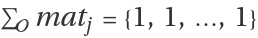.
Now define a graph whose vertices are options and whose edges indicate pairwise disjunction. Cliques in the graph represent maximal sets of disjoint options, which can easily be tested for the exact cover property once they are found.
FindExactCover has a Method option for switching between three distinct algorithms:
 "DancingLinksX" Donald Knuth's algorithm "CliqueToCover" transforms input to a graph and uses FindClique "BranchAndPrune" uses a depth-first search
When using the DancingLinksX method, setting the Heuristic sub-option to either Automatic or "Increment" changes the search order:
The "MRV heuristic" chooses to cover the next item with the minimal number of associated options.
ResourceFunction["FindExactCover"] ignores empty subsets, i.e. zero's rows of the form {0,0,…,0}.

## Examples

### Basic Examples (4)

An IdentityMatrix has only one exact cover:

 In:=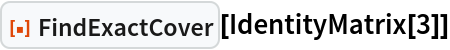Out=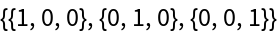List the five set partitions of three objects into disjoint sets:

 In:=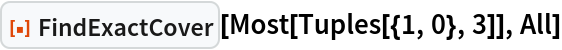Out=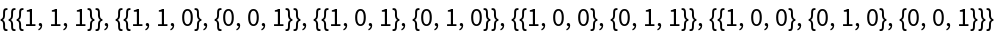Solve an example problem from Wikipedia:

 In:=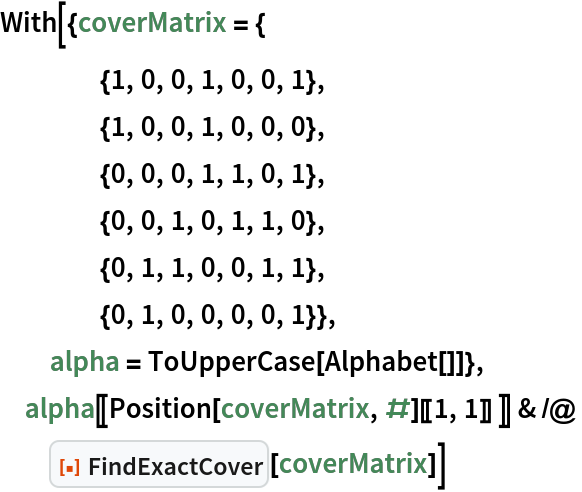Out=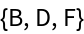Find an exact cover of the columns of a 5×4 matrix:

 In:=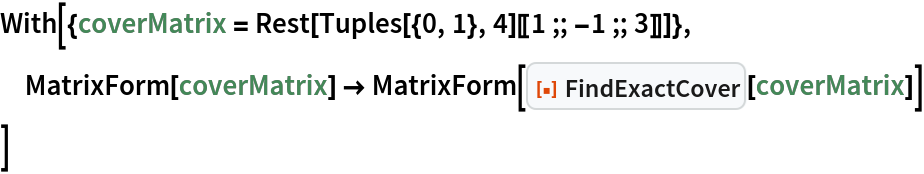Out=Test the row sum property:

 In:=Out=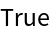Find all exact covers:

 In:=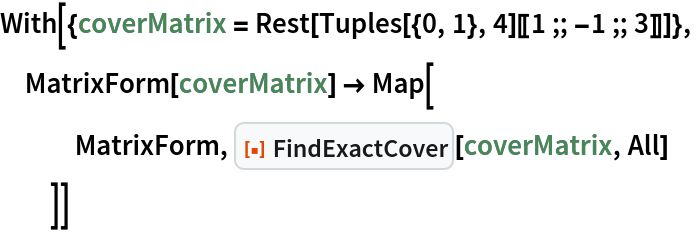Out=### Scope (1)

 In:=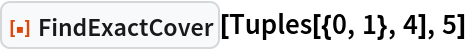Out=### Options (3)

For small simple inputs, setting the "Heuristic" suboption to "Increment" can get results faster:

 In:=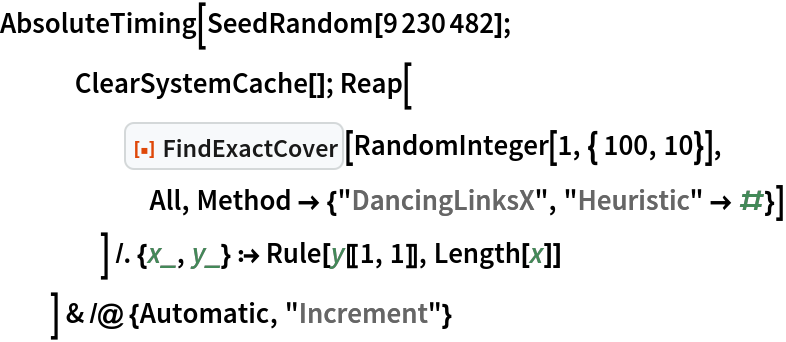Out=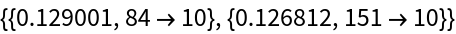The Automatic heuristic can go noticeably faster for more complex inputs:

 In:=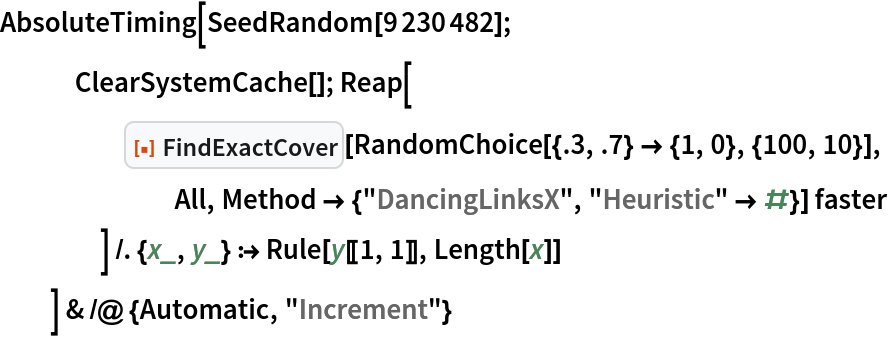Out=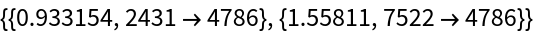But alternative methods can still be faster for relatively small, unstructured inputs:

 In:=Out=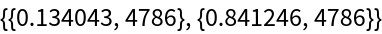### Possible Issues (2)

The default Dancing Links X method should be relatively slow when every valid choice leads to another result:

 In:=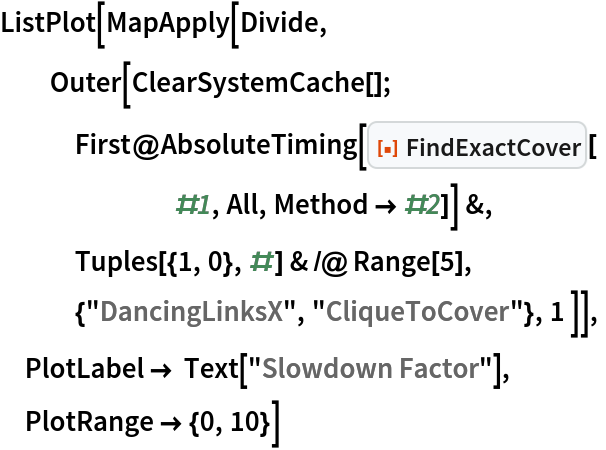Out=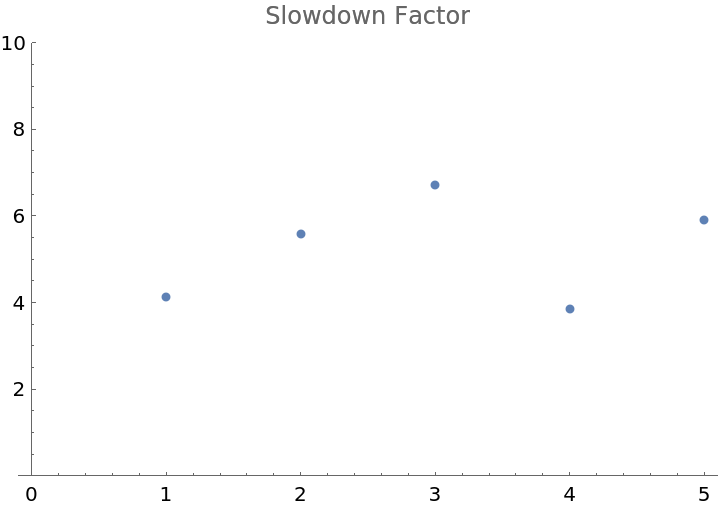Dancing Links X may also be slower in the limit of small inputs:

 In:=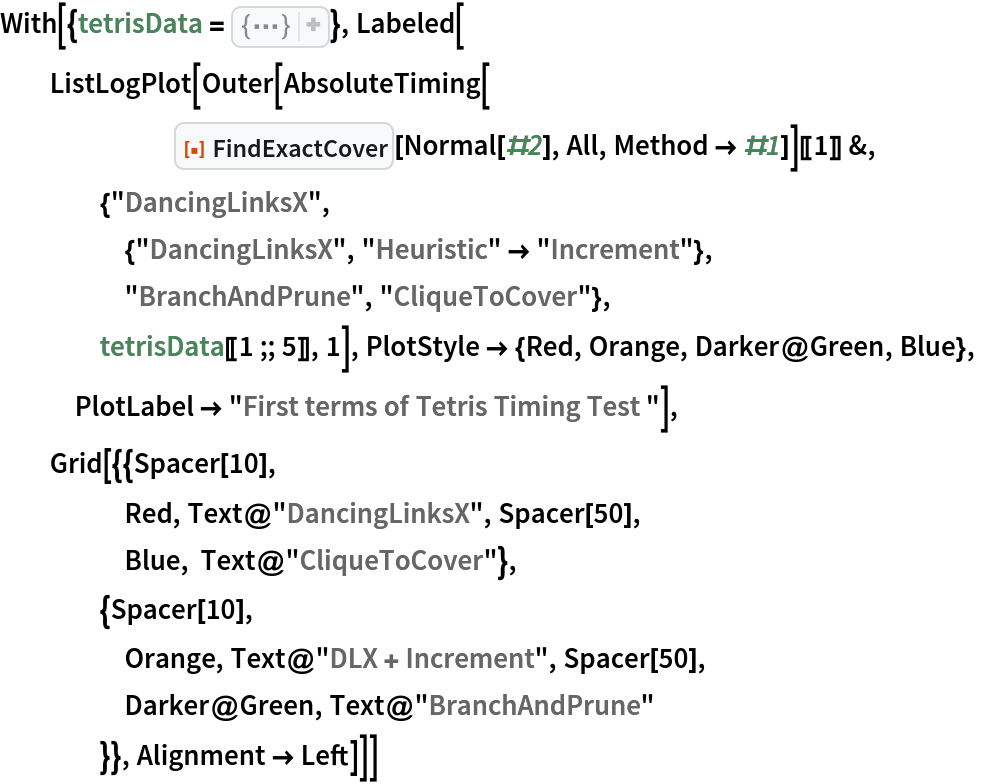Out=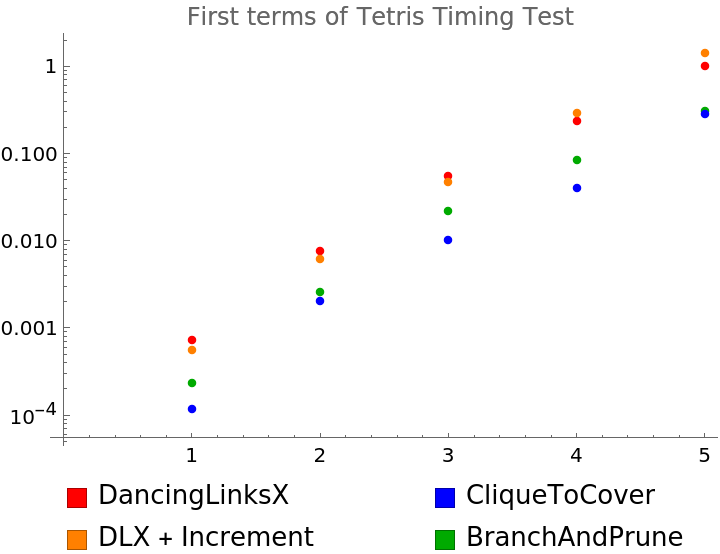But Dancing Links X can have better complexity scaling:

 In:=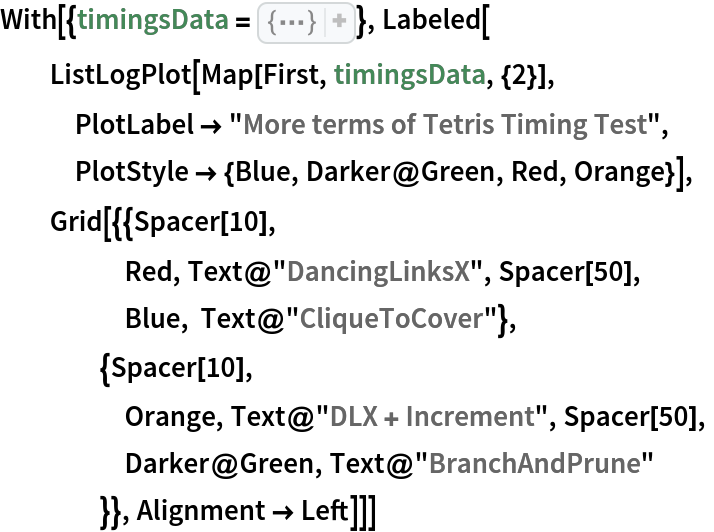Out=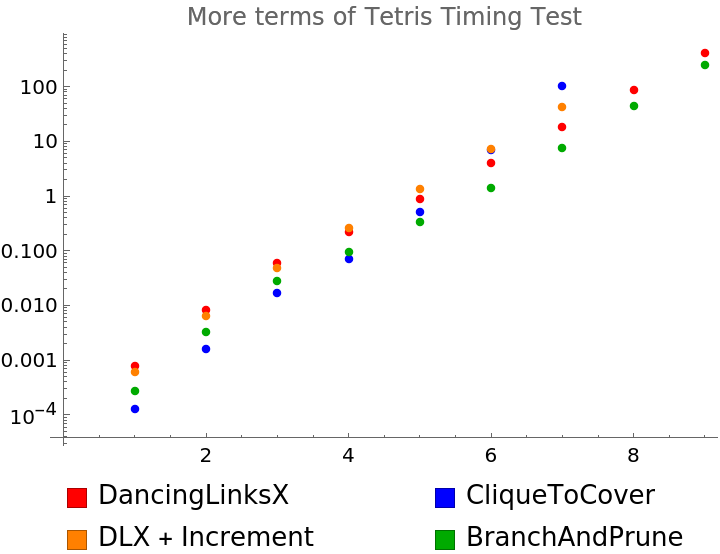### Neat Examples (4)

Find a solution to Scott's pentomino problem:

 In:=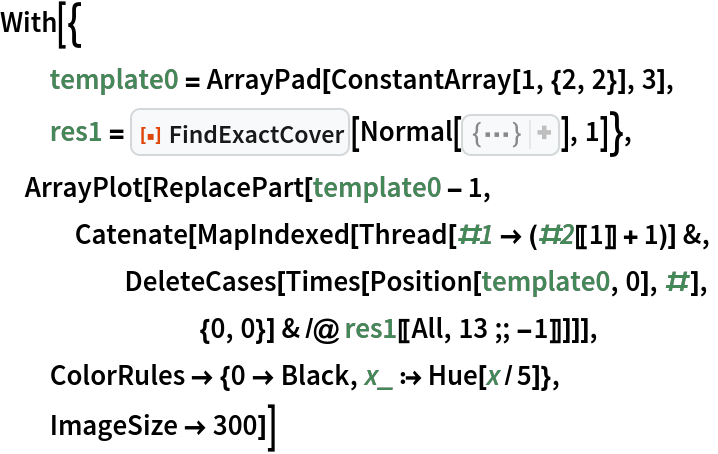Out=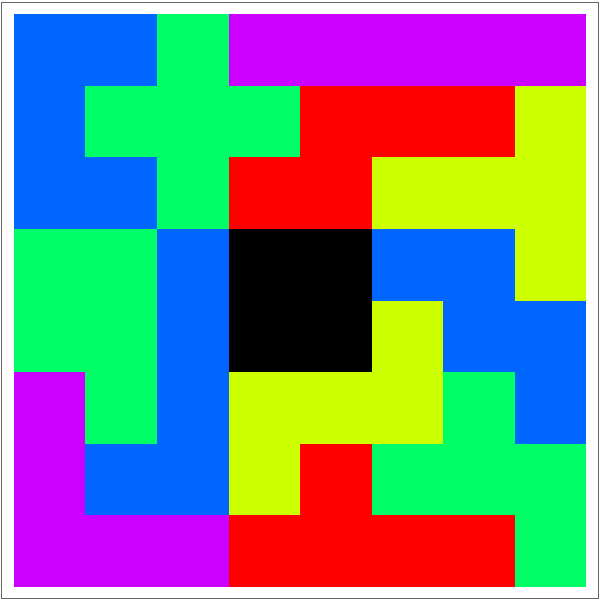Count number of length-n set partitions by enumerating them (cf. OEIS A000110):

 In:=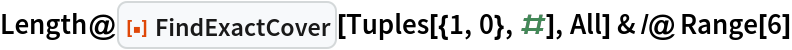Out=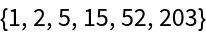Compute the first few terms of the Tetris sequence (cf. OEIS A174248):

 In:=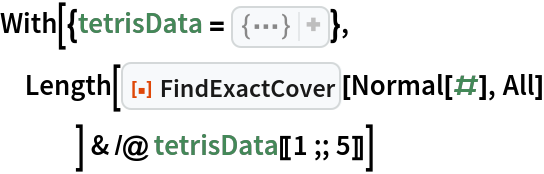Out=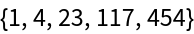Compute the first few terms of the N Queens sequence (cf. OEIS A000170):

 In:=Out=